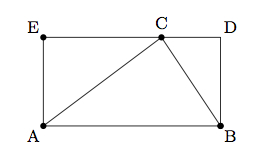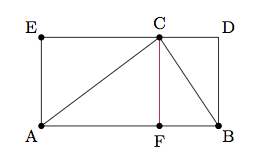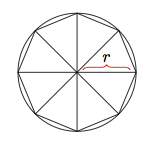# Volume formulas for cylinders and prisms

Alignments to Content Standards: G-GMD.A.1

The volume of a rectangular prism of length $l$, width $w$, and height $h$ is $l \times w \times h$.

1. Use the formula for the volume of a prism with rectangular base to explain why the volume of a right prism with triangular base $T$ and height $h$ is ${\rm Area}(T) \times h$.
2. Use the previous part to show that the volume of a right prism with polygon base $P$ and height $h$ is ${\rm Area}(P) \times h$.
3. Explain why the volume of a cylinder with base $B$ and height $h$ is ${\rm Area}(B) \times h$.

## IM Commentary

The goal of this task is to establish formulas for volumes of right prisms and cylinders. Students encounter these formulas in eighth grade (8.G.9) but arguments based on dissections are introduced in high school. When the side lengths are whole numbers (with a particular choice of units) the formula for prisms comes from the meaning of multiplication and more generally it can be deduced from the whole number case. In solution to part (a), substantial time is taken explaining how to find the area of a polygon (and hence the volume of a prism with polygon base) by triangulating the polygon: this fits well with the ''use dissection arguments'' part of the G-GMD.1 standard. Depending on students' previous experience, some of these results could be taken for granted and then more time can be invested in part (b) of the task.

Students will likely need help understanding the level of rigor expected in these arguments.  For part (a), the argument relies on knowledge of 6.G.1 where the area of polygons is calculated by decomposing into triangles or composing to make rectangles.   In part (b), students need to recognize that every polygon can be dissected into triangles, a fact students will find intuitively obvious after some experimentation, but which is not entirely trivial to carefully justify (although it is easy for convex polygons -- you can explicitly construct the triangles by drawing lines from one given vertex to each non-adjacent vertex).  Similarly, the process for deducing the volume of a cylinder mirrors very closely that for computing the area of circle by approximating with polygons, and then making an informal limit argument as the number of sides of the polygon goes to infinity (as presented for example in https://www.illustrativemathematics.org/illustrations/1567).

## Solution

1. The formula for the volume of a rectangular prism with base $R$ is ${\rm area}(R) \times h$ where $h$ is the height of the prism. If the polygon $P$ which is the base of our prism happens to be a rectangle then we have the desired formula. To establish the formula for other polygon bases, one effective method is to show that the formula is true for any triangular prism. A prism with a polygon as base can then be decomposed into triangular prisms to deduce the general formula.

Below is a picture of a triangle (using the longest side as base) contained in a rectangle:To compare the area of $\triangle ABC$ to the area of rectangle $ABDE$ we add a point $F$ so that $\overleftrightarrow{CF}$ is parallel to $\overleftrightarrow{AE}$ and $\overleftrightarrow{BD}$as seen in the picture below:We can see that $\triangle BFC$ is congruent to $\triangle CDB$ and $\triangle AFC$ is congruent to $\triangle CEA$ since in each case these pairs of triangles are obtained by cutting a rectangle along the diagonal. This means that a prism of height $h$ with $\triangle BFC$ as a base will have the same volume as the prism of height $h$ with $\triangle CDB$ as base. The same holds for the prisms with height $h$ and $\triangle AFC$ and $\triangle CEA$ as bases. We conclude that the volume of a prism with base $\triangle ABC$  and height $h$ is $\frac{1}{2}$ the volume of the prism with base $ABDE$ and height $h$. The volume of the rectangular prism of height $h$ and base $ABDE$ is ${\rm Area}(ABDE) \times h$. Hence the area of the prism with height $h$ and triangle $T$ as base is $\frac{{\rm Area}(ABDE)}{2} \times h$ which is the same as ${\rm Area}(\triangle ABC) \times h$.

2. The case of a prism with polygon base follows from the result for triangles since we can cut the polygonal prism into triangular prisms. For example, below is a picture of a pentagon divided into triangles:The sum of the volumes of the triangular prisms with bases $\triangle APB$, $\triangle BPC$, $\triangle CPD$, $\triangle DPE$, and $\triangle EPA$ is the volume of the prism with base $ABCDE$ and, from the previous paragraph, this is the sum of the areas of the triangles times the height of the prism which is also the area of the pentagon times the height. This dissection argument will work for any prism with a polygon base.

3. We can derive the formula for the volume of a cylinder from the formula for the volume of a prism with polygon base in much the same way that we derive the formula for the area of a circle from the area of approximating polygons. In fact, the argument is identical except that each two dimensional polygon is now a three dimensional prism and as the polygons approximate the circle the prisms approximate the cylinder. Below is a picture showing a regular octagon whose vertices all lie on a given circle of radius of $r$:The volume of the prism with height $h$ and base the regular octagon is ${\rm Area(octagon)} \times h$. More generally, if $P_n$ is a regular $n$-sided polygon inscribed in the circular base of our cylinder, then the volume of the prism with height $h$ and base $P_n$ is ${\rm Area}(P_n) \times h$. As $n$ grows, ${\rm Area}(P_n)$ gets closer and closer to ${\rm Area(Circle)}$. Thus ${\rm Volume(Cylinder)} = {\rm Area(Base)} \times h$.